##### Search### KCSE CLUSTER TEST 14

#### Physics Paper 3

1.

Each candidate requires the following;

- Two new dry cells and cell holder.

- A voltmeter (0.............5V)

- An Ammeter (0.........1.0A)

- A torch Bulb ( for two cells 2.5 – 3.0V)

mounted on a holder. - A Rheostat (0 -50)

- Eight pieces connecting wires.

- At least four crocodile clips.

- A switch. Proceed as follows

a) Connect the apparatus as in the diagram below, such that the Rheostat Controls the brightness of bulb.b) Adjust the Rheostat and record the minimum value of the Voltmeter reading (V0) and the corresponding Ammeter reading (I0) V0 = _________________________V I0 = _________________________ A

c) Adjust the Rheostat again and record the maximum value of the Voltmeter reading (Vx) and the corresponding Ammeter reading (Ix) Vx = _________________________V Ix = _________________________ A

d) Repeat the procedure in (b) above with other values of Voltmeter readings as given in the table below.

Record the corresponding values of the Ammeter reading and repeat the

Table of results.e) Plot the graph p.d (v) against current I( A)g Calculate the estimate value of resistance R0 given by R0 given by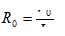g) Calculate the value of resistance Rx given by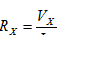h) What does Rx represent?

…………………………………………………………………………………………….

20 marks

2.

a) You are provided with a rectangular glass block, four optical pins, ruler, protractor, soft board, plain paper and cellotape.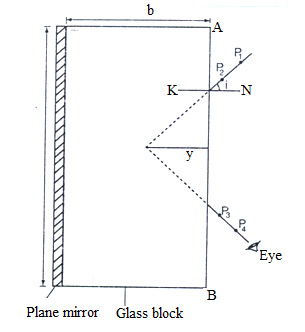i) - Measure and record the breadth, b of the glass block. b =________________cm -

Using cellotape, fix minor on one side (length) of the glass block and trace its outline on the plane paper.

- Draw the normal NK to the side AB and measure angle i =200 from the normal.

- Draw the line representing the incident ray and fix pins P1 and P2 as shown in the figure.

- By observing the images of pins P1 and P2, locate the positions P3 and P4 such that they appear in line with other two pins

. - Join the points P3 and P4 and extend them to intersect line P1P2 produced.

Measure the perpendicular distance, y

- Repeat for angle of incidence i = 300 and record your values in the table below.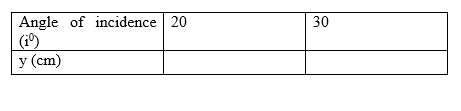Calculate the average value of y the table above ya

iii) Calculate the refractive index of the glass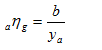5 marks

3.

b) You are provided with the following

- Two metre rule

- An optical pin

- One stand, one boss and a clamp.

- Stop watch.

- A G- clamp.

- A 100g mass.

- A 30g mass

- Some cellotape

Proceed as follows:

a) Using the G–clamp hold the metre rule firmly on the bench such that 90cm of the rule projects from the edge of the bench. (The metre rule should be perpendicular to the edge of the bench and should remain in this position throughouts the experiment) use a piece of cellotape to fix a pin at the protruding end of the metre rule. This will act as a pointer.

Clamp the other metre rule such that the millimeter scale is close to the tip of the pin. Note the reading Y, the initial position of the pointer.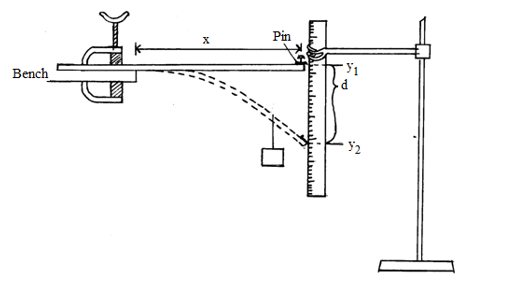) Hang with a loop of thread a 100g mass at a point 5cm from the end of the metre rule. Note Y2 the reading on the scale corresponding to the new position of the pointer. Determine the depression d = ......................(Y1 - Y2). The distance X between the edge of the bench and the position of the mass is now 85cm (90 -5cm)

Repeat the procedure in (c) for other value of X and complete the table below.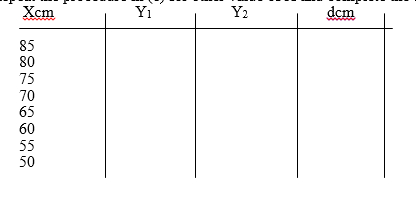e) Remove the mass and note the pointer reading. Hang the 100g mass at the end of the metre rule. Determine the depression d. d = _________________________ cm

Remove the mass f) Now hang a 130g mass on the rule using a loop of thread and adjust its position along the metre rule until the depression is equal to d. Record the value of X at this point

) Displace the end of the rule slightly downwards and release it so that it makes vertical oscillations. Time 20 oscillations and determine periodic time Time (t) for 20 oscillation _________________________s Periodic time T _________________________________ s h) i) Plot a graph of d (y- axis ) against x. ii) Determine the slope S of the graph (2mks) iii) Determine the constant K given that

15 marks

Back Top